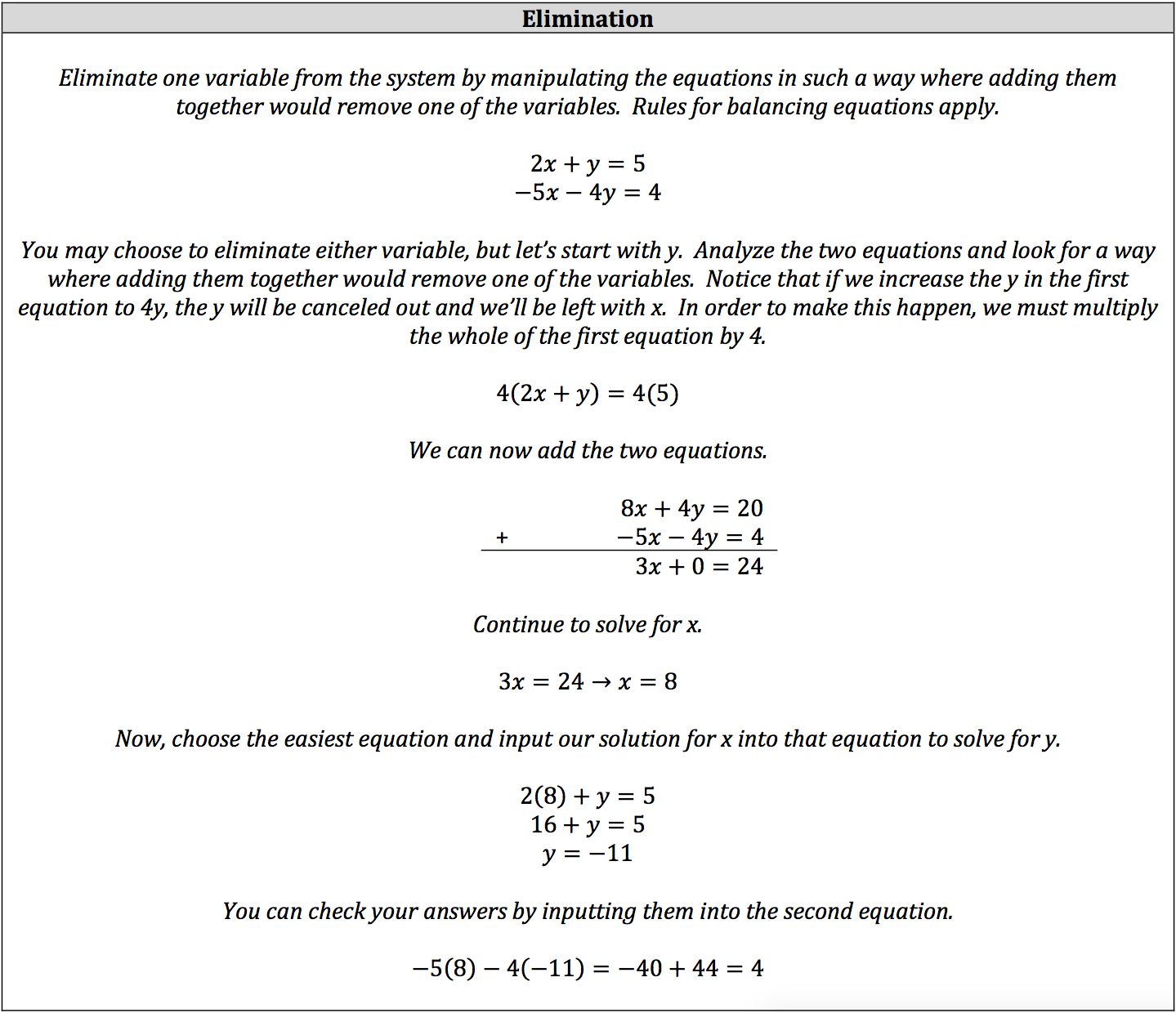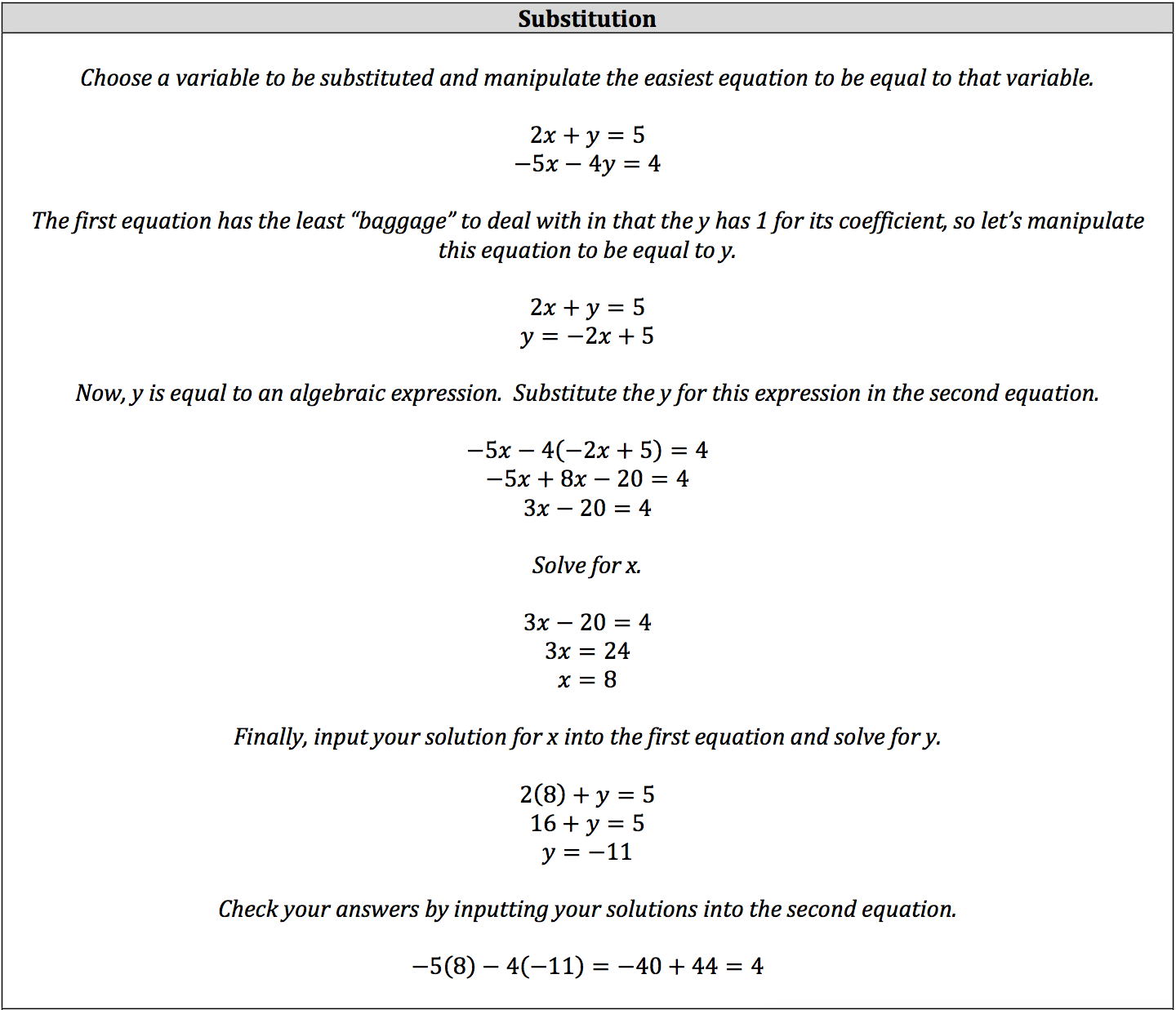So far, you have mostly been solving for a single variable in an equation or placing one variable in terms of the others. However, you will likely face questions asking you to solve for more than one variable, and these questions will likely be about linear equations.

A linear equation simply refers to an equation that will result in a single straight line on a graph, such as slope‐intercept y = mx + b. To solve for more than one variable, you will be working a system of linear equations.

A system is simply a collection of equations that are worked at the same time and come to the same result. This means the only solution to all equations within the system will be the intersection of all the lines on the graph, or where they share the same point.

You can solve a system through elimination or substitution, though both methods are variations of one another.

### Solving Systems Through Elimination### Solving Systems Through Substitution

When it’s not readily apparent how you should manipulate the equations, you can use substitution.• You will notice that our substitution efforts resulted in the same equation with one variable that our elimination efforts did. The only difference as that the 20 was already added to the other side in our elimination efforts.
• When choosing the easiest equation for substitution, focus on which equation would have the least amount of math to perform in setting the equation equal to the variable being substituted.